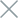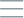• Home
• Tags
• Series
•# Cloud Search Algorithm in C++ - Explanation, Example and Code

The Cloud Search algorithm is a search method that involves generating a large set of random solutions, often referred to as the "cloud," and then searching for the best solutions within this set. This approach is commonly used to find approximate solutions for complex problems when no specific guidance is available.

## How It Works

1. Cloud Initialization: Create a large set of random solutions (the cloud).
2. Evaluation: Evaluate the quality of each solution in the cloud based on the objective function or evaluation criteria.
3. Selection: Select a subset of the best solutions from the cloud based on probabilities or selection criteria.
4. Improvement: Improve the quality of solutions in the cloud by applying transformations or optimizations.
5. Iteration: Repeat steps 2 to 4 until a satisfactory result is achieved or a predefined number of iterations is reached.

## Example: Cloud Search for the Traveling Salesman Problem

Consider the Traveling Salesman Problem (TSP), where the goal is to find the shortest Hamiltonian cycle that visits all cities. The Cloud Search method can generate a large number of random Hamiltonian cycles, then select the cycle with the lowest cost.

## Code Example in C++

``````#include <iostream>
#include <vector>
#include <algorithm>
#include <ctime>
#include <cstdlib>

struct City {
int x;
int y;
};

double calculateDistance(const City& city1, const City& city2) {
return std::sqrt((city1.x - city2.x)*(city1.x - city2.x) + (city1.y - city2.y)*(city1.y - city2.y));
}

double cloudSearchTSP(std::vector<City>& cities, int maxIterations) {
int numCities = cities.size();
double bestDistance = std::numeric_limits<double>::max();

srand(time(0));

for (int i = 0; i < maxIterations; ++i) {
std::random_shuffle(cities.begin(), cities.end());

double totalDistance = 0.0;
for (int j = 0; j < numCities - 1; ++j) {
totalDistance += calculateDistance(cities[j], cities[j + 1]);
}
totalDistance += calculateDistance(cities[numCities - 1], cities);

bestDistance = std::min(bestDistance, totalDistance);
}

return bestDistance;
}

int main() {
std::vector<City> cities = {{0, 0}, {1, 2}, {3, 1}, {4, 3}, {2, 4}};
int maxIterations = 1000;
double shortestDistance = cloudSearchTSP(cities, maxIterations);

std::cout << "Shortest distance in TSP: " << shortestDistance << std::endl;

return 0;
}
``````

In this example, we use the Cloud Search method to solve the TSP. We generate a large number of random Hamiltonian cycles by shuffling the cities randomly, then calculate the cost for each cycle and select the cycle with the lowest cost.